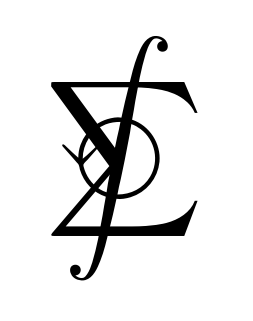On this webpage you will find my solutions to the sixth edition of "Vector Calculus" by Jerrold E. Marsden and Anthony Tromba. Here is a link to the book's page on amazon.com. If you find my work useful, please consider making a donation. Thank you.

• Chapter 1: The Geometry of Euclidean Space
• Section 1.1: Vectors in Two- and Three-Dimensional Space
• Section 1.2: The Inner Product, Length, and Distance
• Section 1.3: Matrices, Determinants, and the Cross Product
• Section 1.4: Cylindrical and Spherical Coordinates
• Section 1.5: n-Dimensional Euclidean Space

• Chapter 2: Differentiation
• Section 2.1: The Geometry of Real-Valued Functions
• Section 2.2: Limits and Continuity
• Section 2.3: Differentiation
• Section 2.4: Introduction to Paths and Curves
• Section 2.5: Properties of the Derivative
• Section 2.6: Gradients and Directional Derivatives

• Chapter 3: Higher-Order Derivatives: Maxima and Minima
• Section 3.1: Iterated Partial Derivatives
• Section 3.2: Taylor's Theorem
• Section 3.3: Extrema of Real-Valued Functions
• Section 3.4: Constrained Extrema and Lagrange Multipliers
• Section 3.5: The Implicit Function Theorem (Optional)
• Chapter 4: Vector-Valued Functions
• Section 4.1: Acceleration and Newton's Second Law
• Section 4.2: Arc Length
• Section 4.3: Vector Fields
• Section 4.4: Divergence and Curl

• Chapter 5: Double and Triple Integrals
• Section 5.1: Introduction
• Section 5.2: The Double Integral Over a Rectangle
• Section 5.3: The Double Integral Over More General Regions
• Section 5.4: Changing the Order of Integration
• Section 5.5: The Triple Integral

• Chapter 6: The Change of Variables Formula and Applications of Integration
• Section 6.1: The Geometry of Maps from R2 to R2
• Section 6.2: The Change of Variables Theorem
• Section 6.3: Applications
• Section 6.4: Improper Integrals (Optional)
• Chapter 7: Integrals Over Paths and Surfaces
• Section 7.1: The Path Integral
• Section 7.2: Line Integrals
• Section 7.3: Parametrized Surfaces
• Section 7.4: Area of a Surface
• Section 7.5: Integrals of Scalar Functions Over Surfaces
• Section 7.6: Surface Integrals of Vector Fields
• Section 7.7: Applications of Differential Geometry, Physics, and Forms of Life

• Chapter 8: The Integral Theorems of Vector Analysis
• Section 8.1: Green's Theorem
• Section 8.2: Stokes' Theorem
• Section 8.3: Conservative Fields
• Section 8.4: Gauss' Theorem
• Section 8.5: Differential Forms
Section 1.4 Section 1.5 Chapter 1 Review
Exercise 1 Exercise 1 Exercise 1 Exercise 26 Exercise 51
Exercise 2 Exercise 2 Exercise 2 Exercise 27 Exercise 52
Exercise 3 Exercise 3 Exercise 3 Exercise 28 Exercise 53
Exercise 4 Exercise 4 Exercise 4 Exercise 29 Exercise 54
Exercise 5 Exercise 5 Exercise 5 Exercise 30 Exercise 55
Exercise 6 Exercise 6 Exercise 6 Exercise 31 Exercise 56
Exercise 7 Exercise 7 Exercise 7 Exercise 32 Exercise 57
Exercise 8 Exercise 8 Exercise 8 Exercise 33
Exercise 9 Exercise 9 Exercise 9 Exercise 34
Exercise 10 Exercise 10 Exercise 10 Exercise 35
Exercise 11 Exercise 11 Exercise 11 Exercise 36
Exercise 12 Exercise 12 Exercise 12 Exercise 37
Exercise 13 Exercise 13 Exercise 13 Exercise 38
Exercise 14 Exercise 14 Exercise 14 Exercise 39
Exercise 15 Exercise 15 Exercise 15 Exercise 40
Exercise 16 Exercise 16 Exercise 16 Exercise 41
Exercise 17 Exercise 17 Exercise 17 Exercise 42
Exercise 18 Exercise 18 Exercise 18 Exercise 43
Exercise 19 Exercise 19 Exercise 19 Exercise 44
Exercise 20 Exercise 20 Exercise 20 Exercise 45
Exercise 21 Exercise 21 Exercise 21 Exercise 46
Exercise 22 Exercise 22 Exercise 22 Exercise 47
Exercise 23 Exercise 23 Exercise 48
Exercise 24 Exercise 24 Exercise 49
Exercise 25 Exercise 50